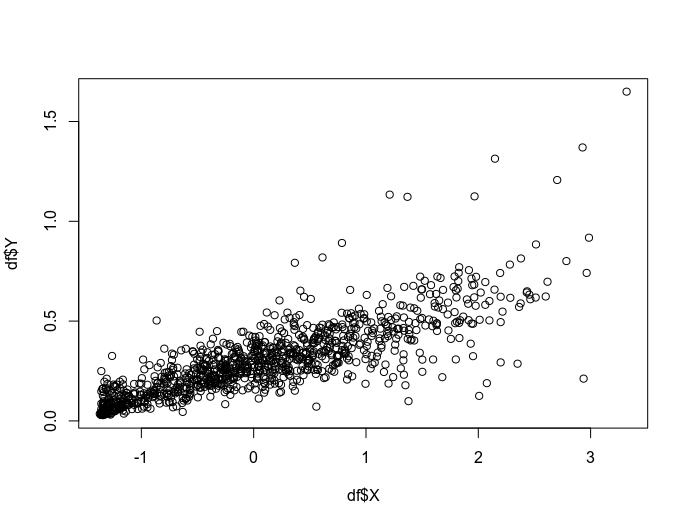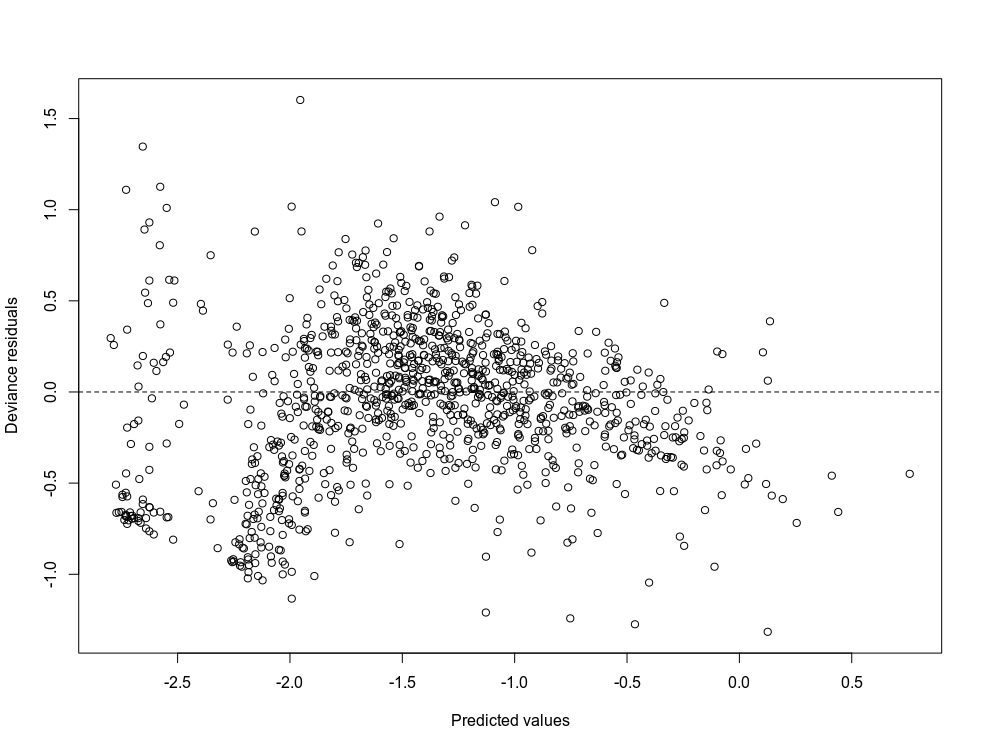# #StackBounty: #r #mixed-model #lme4-nlme #glmm #gamma-distribution What can Ido if I get patterns in residuals vs predicted values usin…

### Bounty: 50

I want to model a response variable (`y`) as a function of two explanatory variables (`x` and `z`). `y` are concentration measures of a physiological parameter of the human body with a worldwide accepted method (method `A`). `x` is a novel method (method `B`) that might be alternative since it is much cheaper. However, it is thought that method `B` is more or less accurate depending on the time (`z`) that the equipment needed is used. So, my goal is to test the significance of `x` and `z` to accurately measure `y`. I have 6 individuals (`ID`: `A`,`B`,`C`,`D`,`E`,`F`). Below I show the relationship between `x` and `y`:Given that my response variable has a sharp non-normal distribution, that the variance increases as `x` increases, and that I have several individuals but I am not interested in differences among individuals, I though on GLMM with a GAMMA distribution to test the significant effect of `x` and `z` for explaining `y`.First, I scaled `x` and `z` since I have read that for interactions is advisable to scale to reduce collinearity between `x` and `z`. Then, I run GLMMs with a gamma distribution and using the log link.

``````df$$X <- scale(df$$X)
df$$Y <- scale(df$$Y)

## Setting random structure ####
mod1<-glmer(Y~ 1 + (1|ID),data = df, family=Gamma(link=log))
mod2<-glmer(Y~ 1 + (X|ID),data = df, family=Gamma(link=log))
AIC(mod1,mod2)

## Setting fixed structure ####
mod3<-glmer(Y~X + Z + (X|ID),data = df, family=Gamma(link=log), control = glmerControl(optimizer ="Nelder_Mead"))
mod4<-glmer(Y~X + X:Z +  (X|ID),data = df, family=Gamma(link=log), control = glmerControl(optimizer ="Nelder_Mead"))

r.squaredGLMM(mod4)[1,c(1,2)]

mod.list <- list(mod1,mod2,mod3,mod4)
model.sel<-model.sel(mod.list, rank="AIC",extra=c(r2=function(x) round(r.squaredGLMM(x)[1,c(1,2)],2)))

``````

To test if `x` and `z` are significant I compared models by AIC.

``````model.sel

Model selection table
(Int)      X       Z     X:Z r2.R2m r2.R2c df   logLik     AIC delta weight
4 -1.300 0.7530         0.04838   0.75   0.78  7 1014.235 -2014.5  0.00  0.923
3 -1.293 0.7341 0.02748           0.74   0.77  7 1011.364 -2008.7  5.74  0.052
2 -1.297 0.7390                   0.74   0.77  6 1009.596 -2007.2  7.28  0.024
1 -1.627                          0.00   0.46  5 1003.959 -1997.9 16.55  0.000
Models ranked by AIC(x)
Random terms (all models):
‘X | ID’
``````

My problem comes when I observe the residual patterns vs predicted values since I think there are clear patterns. Here I don’t show the distribution of the residuals since if I understand correctly, a normal distribution of them is not needed. Alternatively, I haven’t transformed `x` nor `z` since if I understood correctly if I use a GLMM it does not take too much sense to make transformations.Does anyone know what I could do? Any proposal? I did not share the data because is too long (n=1079).

``````head <- read.table(header=TRUE, text="
ID           Z          X          Y
1   A -2.06857299  0.0999264 0.10303660
2   A  0.47766952 -1.5716850 0.06905438
3   A -0.08816215 -1.5873427 0.04125269
4   A -1.36128341  0.2356783 0.27105239
5   A -2.21003091  2.7351100 0.13510265
6   A -2.06857299 -1.5503906 0.04370665
7   A -1.92711507 -1.0819135 0.16452563
8   A  0.19475368 -1.2214479 0.08038283
9   A  1.18495910 -1.4283572 0.04704274
10  A  0.76058535 -1.3846645 0.07481843
11  A  1.46787493 -1.4024705 0.07301108
12  A  0.19475368 -0.9608716 0.12025389
13  A  1.46787493 -1.5005769 0.05606175
14  A  1.18495910 -0.6408735 0.16091346
15  A  0.90204327 -0.4098086 0.25047437
16  A  0.76058535 -1.4679905 0.06739064
17  A  0.61912743 -1.4173005 0.05596254
18  A  0.76058535 -1.3374465 0.07334454
19  A  0.90204327 -0.8583222 0.16164041
20  A  0.47766952  0.9304085 0.46551506
")
``````

Tail of my dataframe:

``````tail <- read.table(header=TRUE, text="
ID           Z          X          Y
269  F  0.05329576 -1.5734595 0.03550929
270  F -0.93690965 -1.1399892 0.03945609
271  F -0.08816215 -1.5723113 0.03062313
272  F  0.19475368 -1.5845737 0.03012372
273  F -0.93690965 -1.5058659 0.05277640
274  F  0.61912743 -1.3621286 0.04939641
275  F  0.05329576 -0.8920383 0.13336570
276  F  0.19475368 -1.4702564 0.11916083
277  F  0.19475368 -1.4853866 0.11188576
278  F -0.08816215 -1.3167784 0.12943442
279  F -0.08816215 -1.3261729 0.17835805
280  F -0.08816215 -1.5647956 0.05257086
281  F -1.07836757 -1.0531661 0.10717841
282  F -1.07836757 -1.5897853 0.06021677
283  F -1.64419924 -1.1298259 0.09429196
284  F -1.36128341 -1.5770086 0.03486231
285  F  0.19475368 -1.4635318 0.09377946
286  F  0.76058535 -1.5638153 0.04056188
287  F  0.19475368 -0.6263252 0.23290781
288  F -2.35148882  0.1957513 0.41243622
")
``````

# Procedure followed according to the proposal of JTH

``````mod1<-glmer(Y~ 1 + (X|ID),data = df, family=Gamma(link=log), control = glmerControl(optimizer ="Nelder_Mead"))
mod2<- glmer(Y~ ns(X, 4)  + (X|ID),data = df, family=Gamma(link=log), control = glmerControl(optimizer ="Nelder_Mead"))
mod3<-glmer(Y~ ns(X, 4) + Z + (X|ID),data = df, family=Gamma(link=log), control = glmerControl(optimizer ="Nelder_Mead"))
mod4<-glmer(Y~ X:Z + ns(X, 4) +  (X|ID),data = df, family=Gamma(link=log), control = glmerControl(optimizer ="Nelder_Mead"))
mod5<-glmer(Y~ ns(X, 4):Z +  (X|ID),data = df, family=Gamma(link=log), control = glmerControl(optimizer ="Nelder_Mead"))
AIC(mod1,mod2,mod3,mod4,mod5)
``````

The results are next:

``````r.squaredGLMM(mod4)[1,c(1,2)]

mod.list <- list(mod1,mod2,mod3,mod4)
model.sel<-model.sel(mod.list, rank="AIC",extra=c(r2=function(x) round(r.squaredGLMM(x)[1,c(1,2)],2)))

model.sel

Int ns(X)            Z       Z:X Z:ns(X)  r2m  r2c df   logLik       AIC     delta       weight
4 -2.827029     +              0.1871558         0.65 0.69 10 320.9342 -621.8684   0.00000 1.000000e+00
2 -2.660326     +                                0.48 0.54  9 289.8442 -561.6884  60.17996 8.552394e-14
3 -2.660204     + 0.0001000814                   0.48 0.54 10 289.8443 -559.6886  62.17976 3.146559e-14
5 -2.220731                                    + 0.04 0.72  9 259.3610 -500.7219 121.14643 4.936132e-27
1 -1.377842                                      0.00 0.27  5 246.9520 -483.9039 137.96446 1.100015e-30
``````

The plot of my residuals vs predicted values is this now:

Get this bounty!!!

This site uses Akismet to reduce spam. Learn how your comment data is processed.